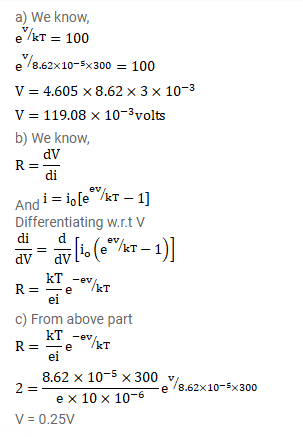# The current-voltage characteristic of an ideal p-n junction diode is given by

Question:

The current-voltage characteristic of an ideal p-n junction diode is given by $\mathrm{i}=\mathrm{i}_{0}\left(\mathrm{e}^{\mathrm{ev} / \mathrm{kT}}-1\right)$

Where the drift current $\mathrm{i}_{0}$ equals $10^{\mu \mathrm{A}}$. A take the temperature $T$ to be $300 \mathrm{~K}$.

(a) Find the voltage $\mathrm{V}_{0}$ for which $\mathrm{e}^{\mathrm{ev} / \mathrm{kT}}=100$. One can neglect the term 1 for voltages greater than this value.

(b) Find an expression for the dynamic resistance of the diode as a function of $\mathrm{V}$ for $\mathrm{V}>\mathrm{V}_{0}$.

(c) Find the voltage for which the dynamic resistance is $0.2 \Omega$.

Solution: